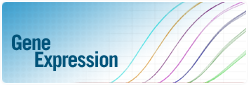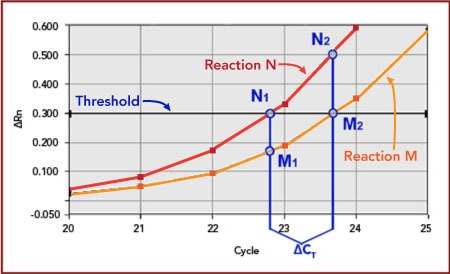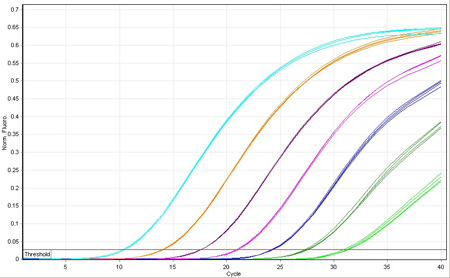## IntroductionSince its invention, PCR has revolutionized the manipulation of gene sequences. With the introduction of quantitative PCR (qPCR) came the ability to measure the expression level of those genes with a precision and sensitivity not afforded previously.

Biosearch supplies the fluorescence-quenched probes and primers that that are the critical component to gene expression analysis. DNA replication triggers fluorescence from the probe so that the kinetics of the amplification can be correlated with initial copy number. Probes also provide additional sequence specificity to ensure that the monitored amplification is truly the gene of interest.

Following is a brief overview of the qPCR mechanism. We outline several different methods of quantification applied to gene expression analysis, but equally applicable toward other uses of copy number determination, for example, loss-of-allele analysis and assaying for viral load.

## Principles of qPCR

PCR products are commonly analyzed after the reaction has completed using double stranded DNA binding dyes like ethidium bromide to reveal the amplified fragments. This end-point analysis is perfectly suited for manipulating the product further but does not provide an indication of the copy number (quantity) before the reaction began.

Combining fluorescent-labeled probes directly into the reaction tube before thermal cycling allows monitoring of amplification in real-time. The signal is designed to remain cloaked until DNA replication through the use of dark quenchers like the Black Hole Quencher® dyes, also attached to the probe and positioned opposite the fluorophore.

Reaction kinetics can be correlated with the initial copy number by monitoring this signal increase. If one reaction amplifies the same target as another but starts from half the number of molecules, then it will consume one additional cycle to achieve the same level of fluorescence. This fluorescence threshold must fall within the exponential phase for the quantitative relationship to be valid. The cycle # where the reaction crosses this threshold is called the Cycle Threshold, or CT value.Figure 1: two qPCR reactions amplify the same sequence but starting from different copy number. If amplification efficiency is known then their relative quantities can be expressed as N/M = E^ΔCT.

The mathematical equation describing exponential amplification is:
N2 = N1 x E^ ΔC

Where N2 is the amount of target molecules after ΔCT cycles, starting from a quantity of N1 and an efficiency of E. A second reaction, M, crosses the same threshold as N1 but with a delay of ΔCT. At this threshold, the quantity of M2 is equal to N1, and can substitute into the above equation: N2 = M2 x E^ΔCT. If efficiency is 100% (E = 2) and a 1 cycle delay observed between the reactions (ΔCT = 1), then N2 is twice the quantity of M2.

## Assay Validation

Gene Expression analysis by qPCR requires a series of techniques, each carefully executed in order to generate a valid result. Because mRNA is inherently unstable, absolute care is needed to ensure that the transcripts have not degraded during extraction and subsequent manipulation. qPCR analysis for gene expression is also sometimes called RT-qPCR since reverse transcription generates the cDNA for amplification. It has been observed that reverse transcription introduces most of the variability in mRNA measurement.

Properties of the reverse transcription reaction in mRNA quantification. Ståhlberg, A., et. al. Clin. Chem. 2004a. 50(3), 509–515.

Once the mRNA has been reverse transcribed into cDNA, several different methods are commonly used to quantify the cDNA, termed absolute and relative quantification. Regardless of the method, a standard template of known concentration is needed to represent the target and validate assay performance. This standard can be a synthetic amplicon, a linearized plasmid, or even a PCR product purified from a previous reaction.Figure 2: a four-fold dilution series of a target standard reveals 2-cycle intervals between CT values, or 100% efficient amplification.

Dilute this standard across a large range of copy number, with each dilution providing a starting point for amplification. Because these quantities are known in advance, the amplification of the series reveals the assay efficiency and sensitivity. Samples of unknown copy number can only be quantified should they fall in the bounds of this validation (within the range of the dilution series).

Amplification efficiency is indicated by the interval of CT values between dilutions. A 10-fold dilution series with intervals of 3.3 cycles indicates 100% efficiency, as determined by the formula: E = 10^(1/k) - 1. k is the dilution interval, also represented by the negative slope of the standard curve.